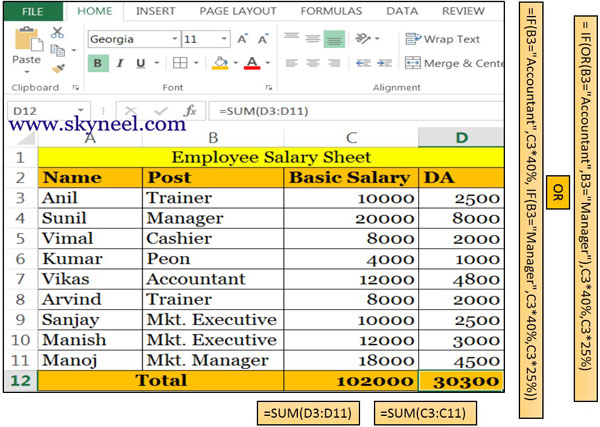# How to use Nested IF function with OR function in MS Excel

Nested IF, AND, OR statements are very popular logical functions in MS Excel.. You can use Nested IF function with OR function in MS Excel

Lot of time we need to apply very popular Logical functions like Nested IF statement for complex calculations, you can also use AND (), OR () functions along with IF () as per requirement. Nested IF function with OR function have proven to be useful in many situations.

### IF Function:

The IF () function is a most popular logical function used in Microsoft Excel. This function allows you to evaluate a situation which has two possible outcomes and calculate a different value for each outcome. However, sometimes you need to work with situations where there are more than two possible outcomes.  You can also you “Nested-IF” function in Excel as per required condition. You can use more than one IF statements is Nested IF function in MS-Excel.

### OR Function:

It is used to check whether any of the condition are True, and returns either TRUE or FALSE. Return FALSE only your conditions is false.  That’s where multiple, or nested, IF functions come in handy. In this tutorial we’ll cover how to use nested IF functions to calculate DA amount based on the post as per given condition

Nested functions use a function as one of the argument of another function. You can nest up to 64 level of functions in Excel 2013. Note: In Excel 2003 you could nest only up to 7 levels under normal working conditions.

E.g. In the example we know how to calculate DA (Dearness Allowance) of an employee in a company as his post. If Post = “Manager” or “Accountant” then DA will be 40% of Basic Salary, otherwise DA will be 25% of Basic Salary.

#### How to use Nested IF with OR Function :

Step 1: Prepare the following sheet.Step 2: Type following formula in cell D3 to calculate DA (Dearness Allowance) as per
given condition:
=IF(OR(B3=”Accountant”,B3=”Manager”),C3*40%,C3*25%)

OR, You can also apply or type another given formula to calculate DA

=IF(B3=”Accountant”,C3*40%, IF(B3=”Manager”,C3*40%,C3*25%))

Step 3: After apply this formula you get the result in cell D3, now drag or copy this formula following range of cells D4:D11.Step 4: Finally you get the following resulted sheet.

I hope you like this article. After reading this article you will be able to easily understand how to use Nested IF function with OR funciton in MS Excel. Give your important suggestions in the comment box. Thanks to all.